GeeksforGeeks App
Open AppBrowser
Continue

# Matrix in R – Arithmetic Operations

Arithmetic operations include addition (+), subtraction (-), multiplication(*), division (/) and modulus(%). In this article we are going to see the matrix creation and arithmetic operations on the matrices in R programming language.

### Approach

• Create first matrix

Syntax:

matrix_name <- matrix(data , nrow = value, ncol = value) .

Parameters:

• data=includes a list/vector of elements passed as data to an matrix.
• nrow= nrow represent the number of  rows  specified.
• ncol= ncol represent the number of  columns  specified.
• Create second matrix
• Apply operation between these matrices
• Display result

Addition yields the sum of the two matrices. Operator used- “+”

Example:

## R

 `# create a vector of elements``vector1=``c``(1,2,3,4,5,6,7,8,9,10,11,12,13,14,15,16)` `# create a matrix with 4* 4 by passing this vector1``matrix1 <- ``matrix``(vector1, nrow = 4, ncol = 4) ` `# display matrix``print``(matrix1)` `# create a vector of elements``vector2=``c``(1,2,3,2,4,5,6,3,4,1,2,7,8,9,4,5)` `# create a matrix with 4* 4 by passing  vector2``matrix2 <- ``matrix``(vector2, nrow = 4, ncol = 4) ` `# display matrix``print``(matrix2)` `# add matrices``print``(matrix1+matrix2)`

Output: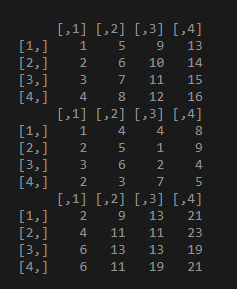### Subtraction

Subtraction yields the difference between two matrices. The operator used: “-“.

Example:

## R

 `# create a vector of elements``vector1=``c``(1,2,3,4,5,6,7,8,9,10,11,12,13,14,15,16)` `# create a matrix with 4* 4 by passing this vector1``matrix1 <- ``matrix``(vector1, nrow = 4, ncol = 4) ` `# display matrix``print``(matrix1)` `# create a vector of elements``vector2=``c``(1,2,3,2,4,5,6,3,4,1,2,7,8,9,4,5)` `# create a matrix with 4* 4 by passing  vector2``matrix2 <- ``matrix``(vector2, nrow = 4, ncol = 4) ` `# display matrix``print``(matrix2)``print``(``" subtraction result"``)` `# subtract matrices``print``(matrix1-matrix2)`

Output: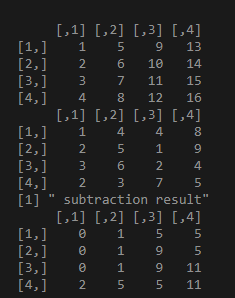### Multiplication

Multiplication results in the multiplication of the elements in a matrix. The operator used: “*”

Example:

## R

 `# create a vector of elements``vector1=``c``(1,2,3,4,5,6,7,8,9,10,11,12,13,14,15,16)` `# create a matrix with 4* 4 by passing this vector1``matrix1 <- ``matrix``(vector1, nrow = 4, ncol = 4) ` `# display matrix``print``(matrix1)` `# create a vector of elements``vector2=``c``(1,2,3,2,4,5,6,3,4,1,2,7,8,9,4,5)` `# create a matrix with 4* 4 by passing  vector2``matrix2 <- ``matrix``(vector2, nrow = 4, ncol = 4) ` `# display matrix``print``(matrix2)``print``(``" multiplication result"``)` `# multiply matrices``print``(matrix1*matrix2)`

Output: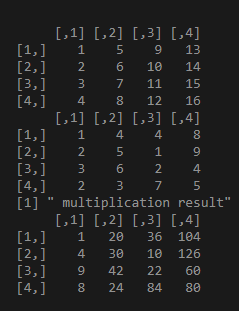### Division

Division is used to divide element by element in the matrices. The operator used : “/”

Example:

## R

 `# create a vector of elements``vector1=``c``(1,2,3,4,5,6,7,8,9,10,11,12,13,14,15,16)` `# create a matrix with 4* 4 by passing this vector1``matrix1 <- ``matrix``(vector1, nrow = 4, ncol = 4) ` `# display matrix``print``(matrix1)` `# create a vector of elements``vector2=``c``(1,2,3,2,4,5,6,3,4,1,2,7,8,9,4,5)` `# create a matrix with 4* 4 by passing  vector2``matrix2 <- ``matrix``(vector2, nrow = 4, ncol = 4) ` `# display matrix``print``(matrix2)``print``(``" Division result"``)` `# divide the matrices``print``(matrix1/matrix2)`

Output: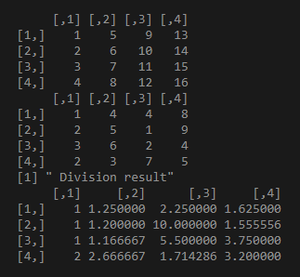### Modulo operation

Modulo returns the remainder of the elements in a matrix. The operator used: %%. The main difference between division and modulo operator is that division returns quotient and modulo returns remainder.

Example:

## R

 `# create a vector of elements``vector1=``c``(1,2,3,4,5,6,7,8,9,10,11,12,13,14,15,16)` `# create a matrix with 4* 4 by passing this vector1``matrix1 <- ``matrix``(vector1, nrow = 4, ncol = 4) ` `# display matrix``print``(matrix1)` `# create a vector of elements``vector2=``c``(1,2,3,2,4,5,6,3,4,1,2,7,8,9,4,5)` `# create a matrix with 4* 4 by passing  vector2``matrix2 <- ``matrix``(vector2, nrow = 4, ncol = 4) ` `# display matrix``print``(matrix2)``print``(``" modulo result"``)` `print``(matrix1%%matrix2)`

Output: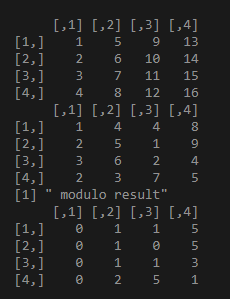My Personal Notes arrow_drop_up